summary:- tips to solve and work out sujiko puzzles for a solution

# sujiko

< back to sujiko start page              see also solving sudoku puzzles and futoshiki puzzles

These Sujiko puzzles are a good example of using pure logic to find the solution. You do not need to be good at maths.

Here are some tips to help you work out a Sujiko puzzle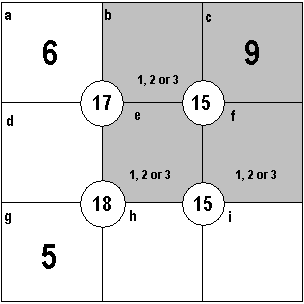### Tip 1.     3 = 6

Look For 3 Squares Totaling 6

At a quick glance, the top right block seems to be a good starting point because 15 - 9 = 6 and we are looking for three different numbers that add up to six - and there are only three that will do that, namely 1 plus 2 plus 3

So we now know that each box b, e and f must be a 1 or a 2 or a 3

It therefore follows that boxes d, h and i cannot be 1, 2 or 3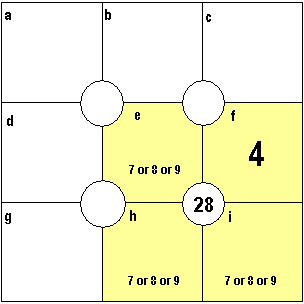### Tip 2.     3 = 24

Look For 3 Squares Totaling 24

The opposite of tip 1 - the lowest possible - is the highest possible for three different numbers nine or under.

9 + 8 + 7 = 24

If a 7 is already solved then a 6 = 23 max and so on down.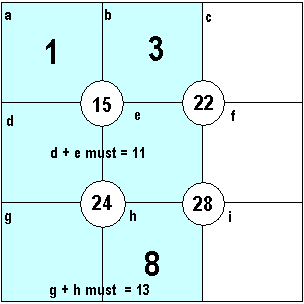### Tip 3.     A Given Pair

If you are given two numbers side by side then you are able to find the sum of the pairs in the row or column under them.

In this example, 1 and 3 are given, therefore...

d + e = 15 -1 -3 = 11

it follows, therefore...

g + h = 24 -11 = 13

The bonus in this example is that we are given that h = 8 and therefore...

g = 13 -8 = 5

This narrows down the possible d and e values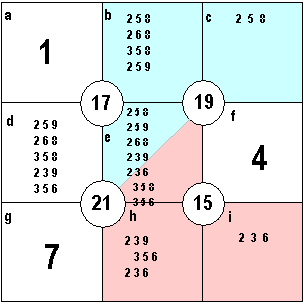### Tip 4.     Common Number

Where two overlapping blocks have three possible numbers for each block, then the square where they overlap will have the value that is common to each block.

With a given number layout like this, square e will overlap with the blue and pink blocks.

Squares b, c and e must be 2 or 5 or 8
Squares e, h and i must be 2 or 3 or 6

Square e cannot be a 3 or 6 to match the blue
Square e cannot be a 5 or 8 to match the pink

Square e must be 2 which is common to all blue and pink blocks (squares b, c, e, h and i)

Once the 2 was found, the solution to this 'extreme' puzzle was easy.

Example of 'lows to highs' using Tip 4 puzzle.

Numbers left to be allocated = 2, 3, 5, 6, 8, 9

Squares b, d and e must total 16 so to start-

2+8+9 = 19 - no
2+6+9 = 17 - no
2+6+8 = 16 - ok
2+5+9 = 16 - ok
2+5+8 = 15 - no
2+5+6 = 14 - no

3+8+9, 3+6+8 and 3+5+9 are too much
3+5+8 = 16 - ok
3+5+6 = 14 - no

5+6+8 or 9 - too much

### Tip 5.     Lows to highs

When finding the possible numbers for each square, start with the lowest number added to the highest two numbers and then work down.

Then pick the second lowest number added to the highest two numbers and work down again.

Very quickly you will reach the 'middle of the available numbers and there is no need to go further as you will only be repeating yourself with the same numbers reversed.

By working logically to a system, though tedious it may be, possible permutations will not be missed.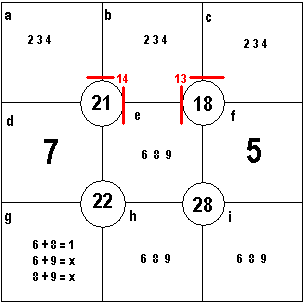I find it helps to write in the numbers left
to place -

1 2 3 4   6   8 9

This was Sujiko #357 in the Daily Telegraph, reproduced with thanks.

### Tip 6.    One More

Look for when there is only one more the difference on a side as marked here in red. Squares b+c+e = 18-5=13 and
Squares a+b+e = 21-7=14 , one more.
Squares b and e must be common so a and c must have a difference of 1

The sujiko puzzle is part solved at the bottom; e+h+i must be 6 or 8 or 9 plus 5 = 28 and running through the possibilities for square g it must be 1 making i = 9 and 6 or 8 for e and h

This leaves only 2, 3 and 4 for the top row and b is common to top left and top right blocks and a is therefore one more than c.

A glance at e will show that if it was 6 the max it could make a top three squares was only 13 so e must = 8

If b was 3 then a and c would be 2 or 4 which is two more so b is not 3. If b is 4 then a and c is 2 and 3 - one more so ok. But, with e = 8 plus b = 4 it makes c=1 and we know g=1 already.

Therefore b must be 2; c must be 3 and a must be 4 - one more

*comments to sudoku@inbox.com* < back to sujiko start page